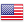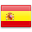## Mathematics

It is the goal of the Lincoln-Marti Schools mathematics department to provide every student with the math background needed to be successful after high school.

This structure in Math Curriculum provides students with the mathematics skills needed for professional careers. The goals of teaching Mathematics at every level is to help all students develop Mathematics power.

The standard course covers:

• General Math (Kindergarten to 7th grade): The student emphasize Numeration and Number Theory, Whole number computation, Fractions and mixed numbers, Decimals, Measurement, Time, Money, Geometry, Problem Solving, Math Reasoning, Estimation, Mental Math, Algebra, Probability and Static, Graphs, Ratio, Proportion, Percent, Patterns, Relations, Functions and Technology.
• Pre-Algebra (8th grade): The purpose and goal is to enable students to successfully bridge the transition from the Arithmetic of the Elementary School to the Algebra for High School.
• Algebra I, Algebra II & Geometry (High School): Provide an understanding of algebraic and geometric concepts.
• Pre-Calculus (12th grade): Provides advanced mathematical concepts, opens with an introduction to a real college student who is utilizing math in his/her chosen major.

SCIENCE LABORATORY

Students will participate in individual and small group activities, where they will develop note taking skills, reading summarizing and dissection techniques.0
0